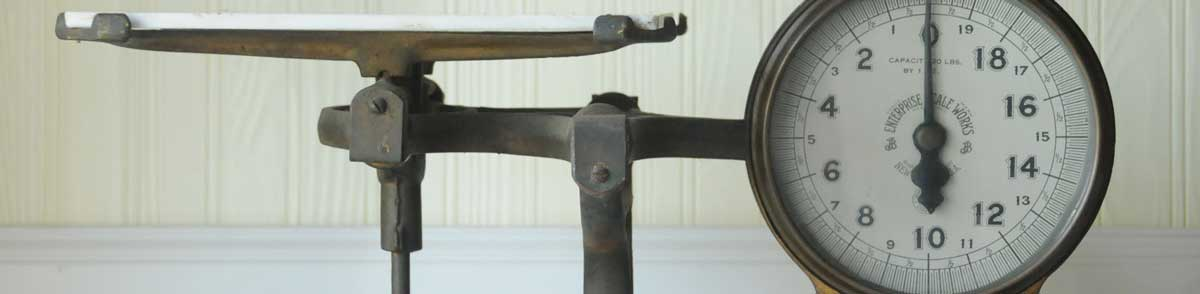# Grams to Milliliters Conversion Calculator

Enter the weight in grams below to calculate the volume in milliliters.

Results in Milliliters:1 g = 1 ml

## How to Convert Grams to MillilitersTo convert a gram measurement to a milliliter measurement, divide the weight by the density of the ingredient or material.

You can use this simple formula to convert:

milliliters = grams ÷ ingredient density

Thus, the weight in milliliters is equal to the grams divided by the density of the ingredient or material.

For example, here's how to convert 5 grams to milliliters for an ingredient with a density of 0.7 g/mL.
5 g = (5 ÷ 0.7) = 7.142857 ml

Grams and milliliters are both commonly used to measure cooking ingredients.

For cooking applications, most chefs suggest measuring dry ingredients by weight rather than volume to improve accuracy in the measurements. The density of dry ingredients can vary for a variety of reasons, such as compaction.

The best way to ensure an accurate conversion is to use a scale. When a scale is not available, a calculator like the one above is a good way to estimate the weight to volume conversion.

## Grams

A gram is the mass/weight equal to 1/1,000 of a kilogram and is roughly equivalent to the mass of one cubic centimeter of water.

The gram, or gramme, is an SI unit of weight in the metric system. Grams can be abbreviated as g; for example, 1 gram can be written as 1 g.

## Milliliters

The milliliter is a unit of volume equal to 1 cubic centimeter, 1/1,000 of a liter, or about 0.061 cubic inches.

The milliliter is an SI unit of volume in the metric system. In the metric system, "milli" is the prefix for 10-3. A milliliter is sometimes also referred to as a millilitre. Milliliters can be abbreviated as ml, and are also sometimes abbreviated as mL or mℓ. For example, 1 milliliter can be written as 1 ml, 1 mL, or 1 mℓ.

Milliliters are sometimes expressed using the abbreviation cc for medical dosage, which is the abbreviation for the cubic centimeter.

## Gram to Milliliter Conversion Table

Gram measurements converted to milliliters for commonly used ingredients.
Weight in Grams: Volume in Milliliters of:
Water Milk Cooking Oil All Purpose Flour Granulated Sugar
1 g 1 ml 0.961538 ml 1.1364 ml 1.8904 ml 1.4286 ml
2 g 2 ml 1.9231 ml 2.2727 ml 3.7807 ml 2.8571 ml
3 g 3 ml 2.8846 ml 3.4091 ml 5.6711 ml 4.2857 ml
4 g 4 ml 3.8462 ml 4.5455 ml 7.5614 ml 5.7143 ml
5 g 5 ml 4.8077 ml 5.6818 ml 9.4518 ml 7.1429 ml
6 g 6 ml 5.7692 ml 6.8182 ml 11.34 ml 8.5714 ml
7 g 7 ml 6.7308 ml 7.9545 ml 13.23 ml 10 ml
8 g 8 ml 7.6923 ml 9.0909 ml 15.12 ml 11.43 ml
9 g 9 ml 8.6538 ml 10.23 ml 17.01 ml 12.86 ml
10 g 10 ml 9.6154 ml 11.36 ml 18.9 ml 14.29 ml
11 g 11 ml 10.58 ml 12.5 ml 20.79 ml 15.71 ml
12 g 12 ml 11.54 ml 13.64 ml 22.68 ml 17.14 ml
13 g 13 ml 12.5 ml 14.77 ml 24.57 ml 18.57 ml
14 g 14 ml 13.46 ml 15.91 ml 26.47 ml 20 ml
15 g 15 ml 14.42 ml 17.05 ml 28.36 ml 21.43 ml
16 g 16 ml 15.38 ml 18.18 ml 30.25 ml 22.86 ml
17 g 17 ml 16.35 ml 19.32 ml 32.14 ml 24.29 ml
18 g 18 ml 17.31 ml 20.45 ml 34.03 ml 25.71 ml
19 g 19 ml 18.27 ml 21.59 ml 35.92 ml 27.14 ml
20 g 20 ml 19.23 ml 22.73 ml 37.81 ml 28.57 ml
21 g 21 ml 20.19 ml 23.86 ml 39.7 ml 30 ml
22 g 22 ml 21.15 ml 25 ml 41.59 ml 31.43 ml
23 g 23 ml 22.12 ml 26.14 ml 43.48 ml 32.86 ml
24 g 24 ml 23.08 ml 27.27 ml 45.37 ml 34.29 ml
25 g 25 ml 24.04 ml 28.41 ml 47.26 ml 35.71 ml
26 g 26 ml 25 ml 29.55 ml 49.15 ml 37.14 ml
27 g 27 ml 25.96 ml 30.68 ml 51.04 ml 38.57 ml
28 g 28 ml 26.92 ml 31.82 ml 52.93 ml 40 ml
29 g 29 ml 27.88 ml 32.95 ml 54.82 ml 41.43 ml
30 g 30 ml 28.85 ml 34.09 ml 56.71 ml 42.86 ml
31 g 31 ml 29.81 ml 35.23 ml 58.6 ml 44.29 ml
32 g 32 ml 30.77 ml 36.36 ml 60.49 ml 45.71 ml
33 g 33 ml 31.73 ml 37.5 ml 62.38 ml 47.14 ml
34 g 34 ml 32.69 ml 38.64 ml 64.27 ml 48.57 ml
35 g 35 ml 33.65 ml 39.77 ml 66.16 ml 50 ml
36 g 36 ml 34.62 ml 40.91 ml 68.05 ml 51.43 ml
37 g 37 ml 35.58 ml 42.05 ml 69.94 ml 52.86 ml
38 g 38 ml 36.54 ml 43.18 ml 71.83 ml 54.29 ml
39 g 39 ml 37.5 ml 44.32 ml 73.72 ml 55.71 ml
40 g 40 ml 38.46 ml 45.45 ml 75.61 ml 57.14 ml

## References

1. National Institute of Standards & Technology, Metric Cooking Resources, https://www.nist.gov/pml/weights-and-measures/metric-cooking-resources
2. National Institute of Standards and Technology, Specifications, Tolerances, and Other Technical Requirements for Weighing and Measuring Devices, Handbook 44 - 2019 Edition, https://nvlpubs.nist.gov/nistpubs/hb/2019/NIST.HB.44-2019.pdf